# GRE Math – Exponent Challenge

A relatively common exponent question on the GRE will ask you to figure out the units digit of some number raised to an exponent, which usually results in a number roughly equivalent to the number of grains of sand in the Sahara, and thus out of reach of the GRE calculator. Take a look at the following problem:

What is the units digit of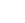?

Before you go scrambling for the google.com calculator, try the following: start small and look for a pattern.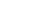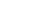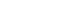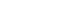First off, don’t let those question marks scare you. Remember, we are only looking for the units digit, so there is no need to know that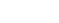, just that it ends in a 9. So when I go to figure out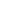, all I have to do is multiply 9 by 3, which is 27, and more importantly, has a units digit of 7.

Now the key to these types of questions is not to multiply all the way up to desired exponent (which would probably eat up the remaining time in the section) but to find a pattern. Notice that the first four numbers, 3, 9, 7, and 1, repeat as soon as you get to. In other words, every exponent that is a multiple of 4 will end in a 1, just asends in a 1. Every number that is one less than an exponent that is a multiple of 4, will end in a 7, just asends in a 7.

Likewise, every even numbered exponent that is not a multiple of 4 will end in 9, just asends in a 9. Therefore,will have a units digit of 9.

Now, let’s take the difficulty level up a notch. Indeed, that is mostly what the GRE quant section does: take familiar concepts and wrap them up in different—and often diabolical—ways.

The following question is very tough, but not tougher than something you might see test day. Take a stab at it!

In the equation,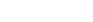, n is a positive integer, and X and Y can be any integer between 1 and 9, meaning that X4 and Y7 are both two-digit integers. What are all the possible values of the units digit of p if p > 0?

[A] 1

[B] 2

[C] 3

[D] 4

[E] 5

[F] 6

[G] 7

[H] 8

[I] 9

## Solution:

The good news is we don’t have to worry at all about the value of X or Y. Remember the (?) above? The value of the tens digit is irrelevant to the units digit, e.g.,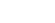, both end in 6.

Therefore, if the value of n is odd, then X4 will always end in 4 (the pattern is 4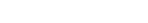…notice how the units digits oscillates between 4 and 6). If n is odd, then the units digit of Y7 will be either 7 or 3. Taking the units digit of 4, from X4 when n is odd, we get 4-7 and 4-3, which will give me 7 and 1.

If n is even, then X4 will always end in 6, and Y7 will end in either 9 or 1 (6 – 9 = 7 and 6 – 1 = 5). Therefore, the possible units digits are 1, 5, and 7, or [A], [E], and [G].

## Author

•Chris Lele is the Principal Curriculum Manager (and vocabulary wizard) at Magoosh. Chris graduated from UCLA with a BA in Psychology and has 20 years of experience in the test prep industry. He's been quoted as a subject expert in many publications, including US News, GMAC, and Business Because. In his time at Magoosh, Chris has taught countless students how to tackle the GRE, GMAT, SAT, ACT, MCAT (CARS), and LSAT exams with confidence. Some of his students have even gone on to get near-perfect scores. You can find Chris on YouTube, LinkedIn, Twitter and Facebook!